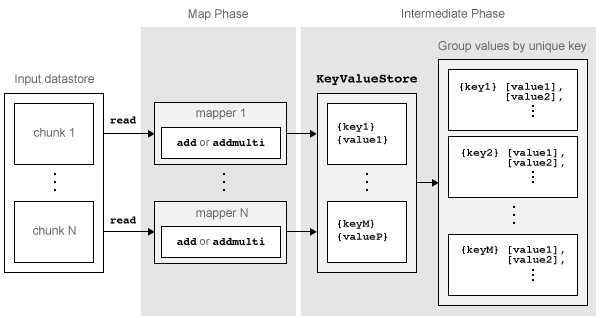## Write a Map Function

### Role of Map Function in MapReduce

`mapreduce` requires both an input map function that receives blocks of data and that outputs intermediate results, and an input reduce function that reads the intermediate results and produces a final result. Thus, it is normal to break up a calculation into two related pieces for the map and reduce functions to fulfill separately. For example, to find the maximum value in a data set, the map function can find the maximum value in each block of input data, and then the reduce function can find the single maximum value among all of the intermediate maxima.

This figure shows the Map phase of the `mapreduce` algorithm.The Map phase of the `mapreduce` algorithm has the following steps:

1. `mapreduce` reads a single block of data using the `read` function on the input datastore, then calls the map function to work on the block.

2. The map function then works on the individual block of data and adds one or more key-value pairs to the intermediate `KeyValueStore` object using the `add` or `addmulti` functions.

3. `mapreduce` repeats this process for each of the blocks of data in the input datastore, so that the total number of calls to the map function is equal to the number of blocks of data. The `ReadSize` property of the datastore determines the number of data blocks.

The Map phase of the `mapreduce` algorithm is complete when the map function processes each of the blocks of data in the input datastore. The result of this phase of the `mapreduce` algorithm is a `KeyValueStore` object that contains all of the key-value pairs added by the map function. After the Map phase, `mapreduce` prepares for the Reduce phase by grouping all the values in the `KeyValueStore` object by unique key.

### Requirements for Map Function

`mapreduce` automatically calls the map function for each block of data in the input datastore. The map function must meet certain basic requirements to run properly during these automatic calls. These requirements collectively ensure the proper movement of data through the Map phase of the `mapreduce` algorithm.

The inputs to the map function are `data`, `info`, and `intermKVStore`:

• `data` and `info` are the result of a call to the `read` function on the input datastore, which `mapreduce` executes automatically before each call to the map function.

• `intermKVStore` is the name of the intermediate `KeyValueStore` object to which the map function needs to add key-value pairs. The `add` and `addmulti` functions use this object name to add key-value pairs. If the map function does not add any key-value pairs to the `intermKVStore` object, then `mapreduce` does not call the reduce function and the resulting datastore is empty.

In addition to these basic requirements for the map function, the key-value pairs added by the map function must also meet these conditions:

1. Keys must be numeric scalars, character vectors, or strings. Numeric keys cannot be `NaN`, complex, logical, or sparse.

2. All keys added by the map function must have the same class.

3. Values can be any MATLAB® object, including all valid MATLAB data types.

Note

The above key-value pair requirements may differ when using other products with `mapreduce`. See the documentation for the appropriate product to get product-specific key-value pair requirements.

### Sample Map Functions

Here are a few illustrative map functions used in `mapreduce` examples.

#### Identity Map Function

A map function that simply returns what `mapreduce` passes to it is called an identity mapper. An identity mapper is useful to take advantage of the grouping of values by unique key before doing calculations in the reduce function. The `identityMapper` mapper file is one of the mappers used in the example Tall Skinny QR (TSQR) Matrix Factorization Using MapReduce.

```function identityMapper(data, info, intermKVStore) % This mapper function simply copies the data and add them to the % intermKVStore as intermediate values. x = data.Value{:,:}; add(intermKVStore,'Identity', x); end```

#### Simple Map Function

One of the simplest examples of a nonidentity mapper is `maxArrivalDelayMapper`, which is the mapper for the example Find Maximum Value with MapReduce. For each chunk of input data, this mapper calculates the maximum arrival delay and adds a key-value pair to the intermediate `KeyValueStore`.

```function maxArrivalDelayMapper (data, info, intermKVStore) partMax = max(data.ArrDelay); add(intermKVStore, 'PartialMaxArrivalDelay',partMax); end```

A more advanced example of a mapper is `statsByGroupMapper`, which is the mapper for the example Compute Summary Statistics by Group Using MapReduce. This mapper uses a nested function to calculate several statistical quantities (count, mean, variance, and so on) for each chunk of input data, and then adds several key-value pairs to the intermediate `KeyValueStore` object. Also, this mapper uses four input arguments, whereas `mapreduce` only accepts a map function with three input arguments. To get around this, pass in the extra parameter using an anonymous function during the call to `mapreduce`, as outlined in the example.

```function statsByGroupMapper(data, ~, intermKVStore, groupVarName) % Data is a n-by-3 table. Remove missing values first delays = data.ArrDelay; groups = data.(groupVarName); notNaN =~isnan(delays); groups = groups(notNaN); delays = delays(notNaN); % Find the unique group levels in this chunk [intermKeys,~,idx] = unique(groups, 'stable'); % Group delays by idx and apply @grpstatsfun function to each group intermVals = accumarray(idx,delays,size(intermKeys),@grpstatsfun); addmulti(intermKVStore,intermKeys,intermVals); function out = grpstatsfun(x) n = length(x); % count m = sum(x)/n; % mean v = sum((x-m).^2)/n; % variance s = sum((x-m).^3)/n; % skewness without normalization k = sum((x-m).^4)/n; % kurtosis without normalization out = {[n, m, v, s, k]}; end end```

#### More Map Functions

For more information about common programming patterns in map or reduce functions, see Build Effective Algorithms with MapReduce.